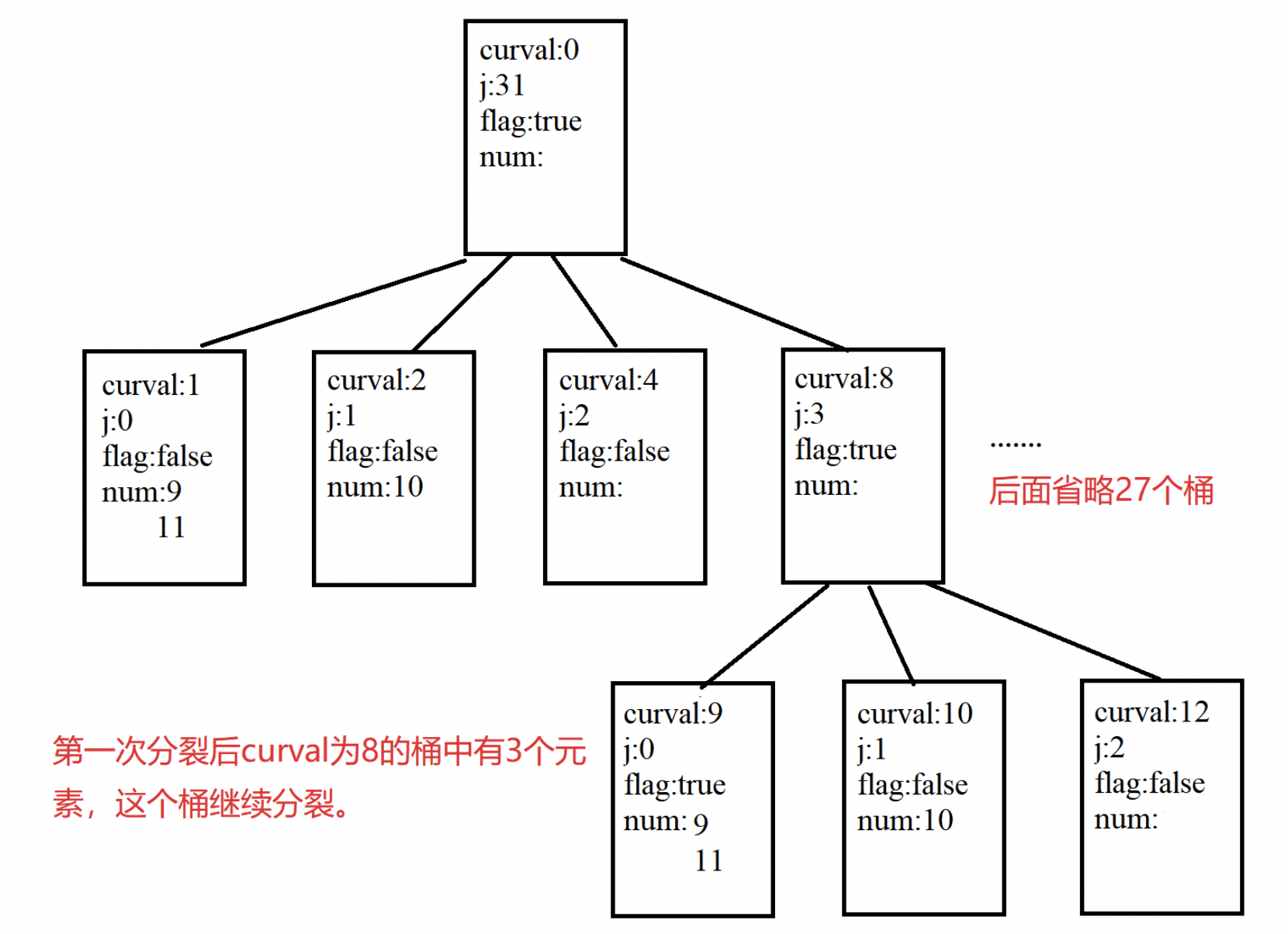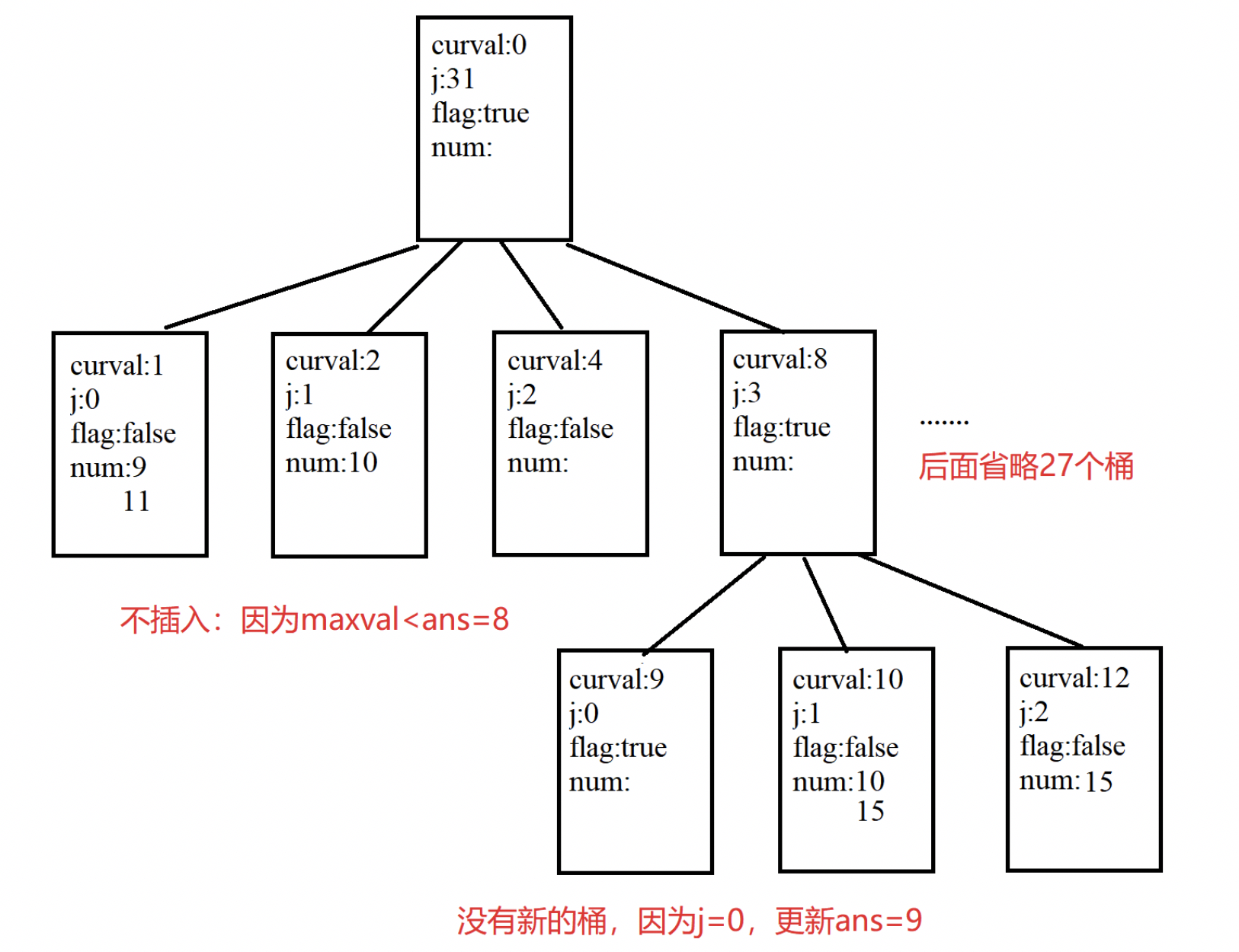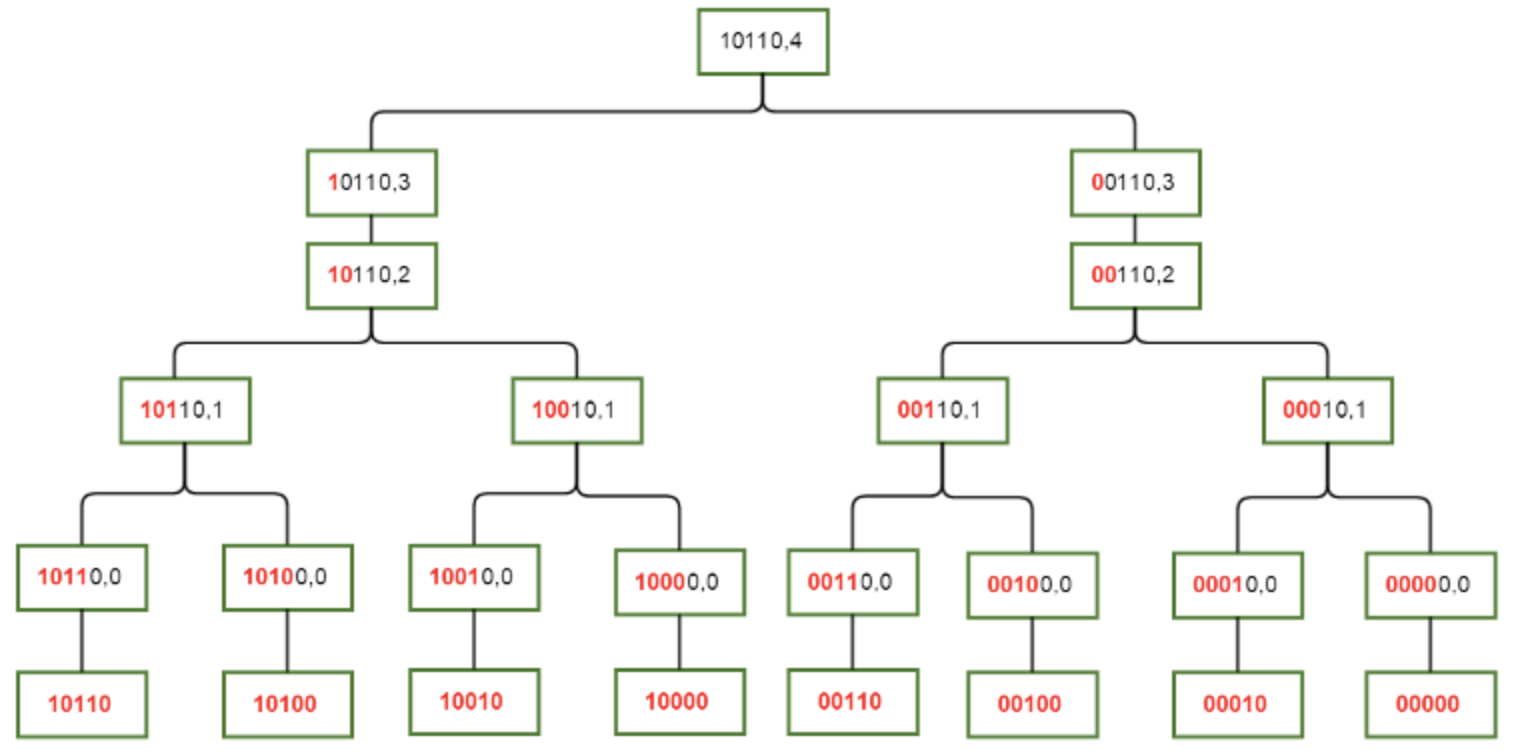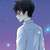# 2019.9 月赛题解

#### 花絮

• 这次的题目，码量很少。
• 久违的温暖场了吧。全面开花。
• 不过没有人 AK ，还是有点诧异。
• 事实上，Cuber QQ 并没有参加军训，所以他每天都在划水。
• 事实上，Cuber QQ 的唱跳大家并没有领略过。
• 事实上，丽娃河没有鸭子，荷花倒是不少。
• 好像我接锅月赛以后， Monthly 变成了 Every Two Months ，说不定以后变成 Quarterly 了也说不定。# Tag Idea Developer Tester
A 博弈 cs2017 Xiejiadong Kilo_5723 10175101214
C 签到 Weaver_zhu Weaver_zhu 10175101214 oxx1108
D 数学 Kilo_5723 Kilo_5723 10175101214 Grunt
E DP Grunt Grunt 10175101214 Xiejiadong

### Problem A

##### First Solved：ustczml

10175101214 ： 这题博弈和我以往做的不太一样，挺有意思的。

### Problem C

##### First Solved：ReTleWa

oxx1108 ： 感觉这题的坑，挖的有些许生硬。

### Problem D

##### First Solved：SuperSodaSea

Grunt ： 这题知乎上有答案。

Kilo_5723 ： 当时是看到了 $4$ 只鸭子的题目，发现可以推广，就拿过来了。

### Problem E

##### 解法 1 ：
###### Part One

• 元素 $num$ ：表示桶里面放的元素（最多 $3$ 个，若一个桶中有 $3$ 个元素时，则表示可以用 $curval$ 更新答案了，此时需要将桶分裂）。
• 指针 $point$ ：记录桶分裂后，新的桶的地址。
• 标识 $flag$ ：标记桶是否分裂过。

• 插入 $insert$ ：插入一个元素，若插入导致桶溢出（达到 $3$ 个数），则桶分裂。
• 分裂 $split$ ：一个桶分裂成 $j$ 个桶，分裂后需要重新分配桶中的三个元素。

###### Part Two 案例分析

1、插入 $9$ 和 $10$ ：直接插入

curval:0
j:31
flag=false
num:9
10


2、插入 $11$ ：由于桶中元素已经满了，所以需要将桶分裂成 $31$ 个桶：其 $curval$ 分别为： $1，2，4，8，…$ ； $j$ 分别为 $0，1，2，3，…$ 。表示含义枚举下一个 $1$ 填在哪里，若下一个 $1$ 填为第 $0$ 位，则答案变成 $1$ ，此时所有位都确定（因为下一位填在第 $0$ 位意味着第 $1-31$ 位都不可能填），后面的桶类似。现在考虑将 $9 10 11$ 重新分配成 $31$ 个新的桶中：由于 $9$ 的二进制是 $1001$ ，所以对 $curval$ 为 $1$ 和 $8$ 的桶有贡献，所以插入在这两个桶中，类似 $10$ 放入 $curval$ 为 $2$ 和 $8$ 的桶， $11$ 放入 $curval$ 为 $1，2，8$ 的桶中（注意：若插入过程新桶也满了，如例子中 $curval$ 为 $8$ 的桶，则递归这个过程）。（此时 $ans=8$ ）3、插入 $15$ （此时 $ans=9$ ）###### Part Three 复杂度证明

1、插入过程：如果插入一个数桶没有满则直接插入，若需要桶分裂，假设分离了 $j=k$ 的桶，则所以 $j < k$ 的桶都无需继续考虑，因为 $maxval < ans$ ，而每一次的分裂需要确定的二进制位数至少减少 $1$ ，将原来桶中的 $3$ 个数重新分配需要 $O(log(n))$ 的复杂度，而重新分配过程中最多继续分裂一个桶，所以插入一个数最多分裂 $log(n)$ 个桶，所以插入复杂度为 $O(log(n)^2)$ 。

2、查询过程：动态维护 $ans$ ，查询 $O(1)$ 。

##### 解法二### 恭喜 cy1999 ， Manari ， Gzhu_ConanYu 冠亚季军。